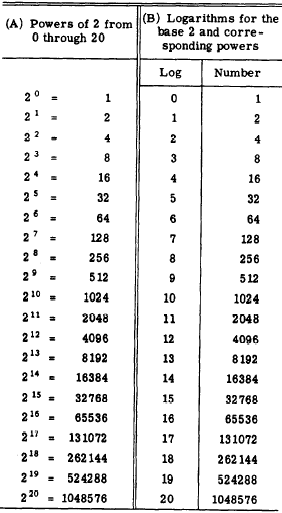CHAPTER 8 - LOGARITHMS AND THE SLIDE RULECustom SearchCHAPTER 8 LOGARITHMS AND THE SLIDE RULE Logarithms ‘represent a specialized use of exponents. By means of logarithms, computation with large masses of data can be greatly simplified. For example, when logarithms are used, the process of multiplication is replaced by simple addition and division is replaced by subtraction. Raising to a power by means of logarithms is done in a single multiplication, and extracting a root reduces to simple division. DEFINITIONS In the expression 23 = 8, the number 2 is the base (not to be confused with the base of the number system), and 3 is the exponent which must be used with the base to produce the number 8. The exponent 3 is the logarithm of 8 when the base is 2. This relationship is usually stated as follows: The logarithm of 8 to the base 2 is 3. In general, the logarithm of a number N with respect to a given base is the exponent which must be used with the base to produce N. Table 8-l illustrates this. Table 8-l.-Logarithms with various bases.Table 8-l shows that the logarithmic relationship may be expressed equally well in either of two forma; these are the exponential form and the logarithmic form. Observe, in table 8-1, that the base of a logarithmic expression is indicated by placing a subscript just below and to the right of the abbreviation ‘log." Observe also that the word ‘logarithm" is abbreviated without using a period. The equivalency of the logarithmic and exponential forms may be used to restate the fundamental definition of logarithms in its most useful form, as follows: bX = N implies that logbN = x In words, this definition is stated as follows: If the base b raised to the x power equals N, then x is the logarithm of the number N to the base b. One of the many uses of logarithms may be shown by an example in which the base is 2. Table 8-2 shows the powers of 2 from 0 through 20. Suppose that we wish to use logarithms to multiply the numbers 512 and 256, as follows: From table 8-2, 512 = 29                             256 = 28 Then         512 x 256 = 29 x 28                                     = 217 and from the table again 217 = 131072 It is seen that the problem of multiplication is reduced to the simple addition of the exponents 9 and 8 and finding the corresponding power in the table. Table 8-2 (A) shows the base 2 in the exponential form with its corresponding powers. The actual computation in logarithmic work does not require that we record the exponential form. All that is required is that we add the appropriate exponents and have available a table in which we can look up the number corresponding to the new exponent after adding. Therefore, table 8-2 (B) is adequate for our purpose. Solving the foregoing example by this table, we have the following:     log2 512 = 9     log2 256 = 8 log2 of the product = 17 Therefore, the number we seek is the one in the table whose logarithm is 17. This number is 131,072. In this example, we found the exponents directly, added them since this was a Table 8-2 .-Exponential and logarithmic tables for the base 2.multiplication problem, and located the correspending power. This avoided the unnecessary step of writing the base 2 each time. Practice problems. Use the logarithms in table 8-2 to perform the following multiplication: 1. 64 x 128  2. 1,024 x 256  3. 128 x 4,096 4. 512 x 2,048 Answers: 1. 8,192  2. 262,144  3. 524,288 4. 1,048,576 NATURAL AND COMMON LOGARITHMS Many natural phenomena, such as rates of growth and decay, are most easily described in terms of logarithmic or exponential formulas. Furthermore, the geometric patterns in which certain seeds grow (for example, sunflower seeds) is a logarithmic spiral. These facts explain the name "natural logarithms." Natural logarithms use the base e, which is an irrational number approximately equal to 2.71828. This system is sometimes called the Napierian system of logarithms, in honor of John Napier, who is credited with the invention of logarithms.  To distinguish natural logarithms from other logarithmic systems the abbreviation, In, is sometimes used. When In appears, the base is understood to be e and need not be shown. For example, either log, 45 or In 45 signifies the natural logarithm of 45.Integrated Publishing, Inc. - A (SDVOSB) Service Disabled Veteran Owned Small Business